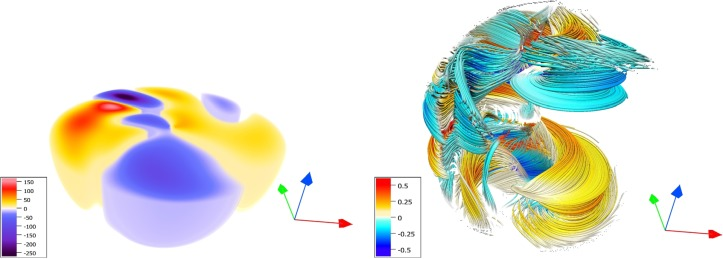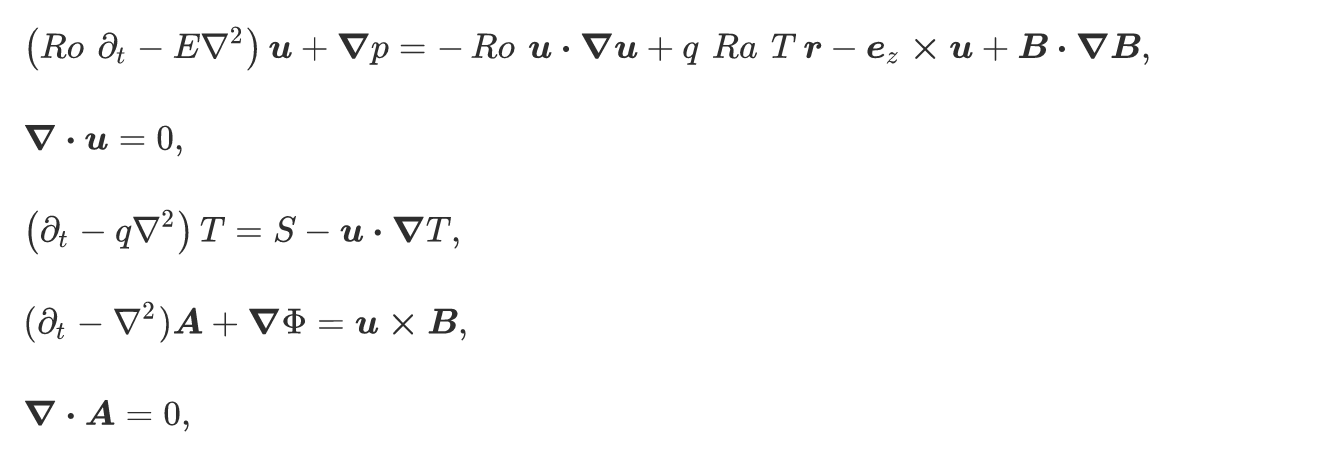# Papers published!

I’m excited to annouce that our two papers on spin-weighted spherical harmonics are finally published in the Journal of Computational Physics X. The first paper covers the mathematical formalism, while the second paper demonstrates its performance in a number of test problems, including a fully convective, rotating dynamo problem:On the right is the radial velocity while the left shows magnetic field lines, visualized using Vapor.

The most significant part of this research, aside from the fact that we have sparse, efficient spectrally accurate spherical basis functions in Dedalus, is that by using spin-weighted spherical harmonics, one can implement equations on the sphere directly in vector form:Previously, one would have to write equations in poloidal-toridal form in order to expand them in spherical harmonics. By constructing spectrally accurate solutions for vector partial differential equations on the sphere, we can now get ready for a lot of exciting research on the sphere!